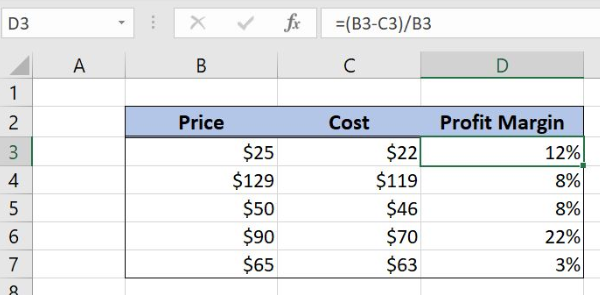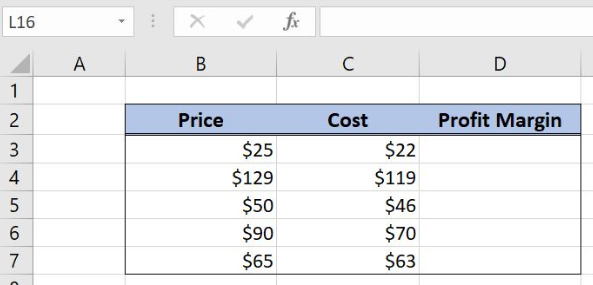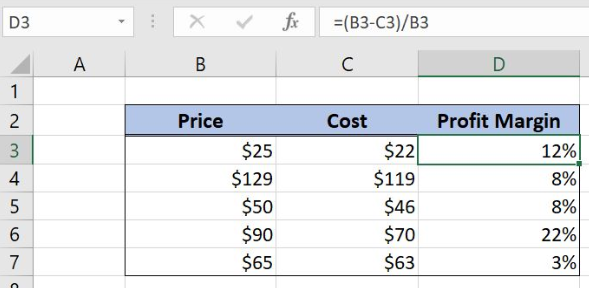Get instant live expert help with Excel or Google Sheets“My Excelchat expert helped me in less than 20 minutes, saving me what would have been 5 hours of work!”

#### Post your problem and you'll get expert help in seconds

Your message must be at least 40 characters
Our professional experts are available now. Your privacy is guaranteed.

# Get profit margin percentage

Excel allows a user to calculate profit margin percentage from a sale price and cost, using the simple formula. The profit margin is calculated as a rate between price and cost difference and price. This step by step tutorial will assist all levels of Excel users in getting a profit margin percentage.Figure 1. The result of the formula

## Syntax of the Formula

The generic formula is:

`=(price - cost)/price`

The parameters of the formula are:

• price – a sale price.
• cost – the cost of the product.

## Setting up Our Data for the Example

Let’s look at the structure of the data we will use. In column B, we have the price and in column C, we have the cost. In column D, we want to calculate a profit margin percentage.Figure 2. Data that we will use in the example

## Calculating the Profit Margin Percentage

In our example, the sale price is \$25 (B3) and the cost is \$22 (C3) Based on these two values, we want to calculate the profit margin percentage in the cell D3.

The formula looks like:

`=(B3 - C3)/B3`

The parameter price is the cell B3, while the cost is in C3.

To apply the formula, we need to follow these steps:

• Select cell D3 and click on it
• Insert the formula: `=(B3 - C3)/B3`
• Press enter
• Drag the formula down to the other cells in the column by clicking and dragging the little “+” icon at the bottom-right of the cell.Figure 3. Calculating the profit margin percentage

The result in the cell D3 is 12%, which is the profit margin for the price of \$25 and the cost \$22.

Most of the time, the problem you will need to solve will be more complex than a simple application of a formula or function. If you want to save hours of research and frustration, try our live Excelchat service! Our Excel Experts are available 24/7 to answer any Excel question you may have. We guarantee a connection within 30 seconds and a customized solution within 20 minutes.

### Did this post not answer your question? Get a solution from connecting with the expert.Another blog reader asked this question today on Excelchat:## Subscribe to Excelchat.coAnother blog reader asked this question today on Excelchat: